# 循环轮转矩阵

## 1914. 循环轮转矩阵 (Medium)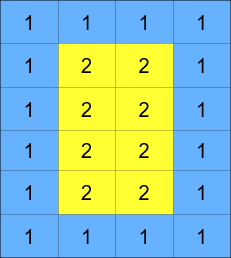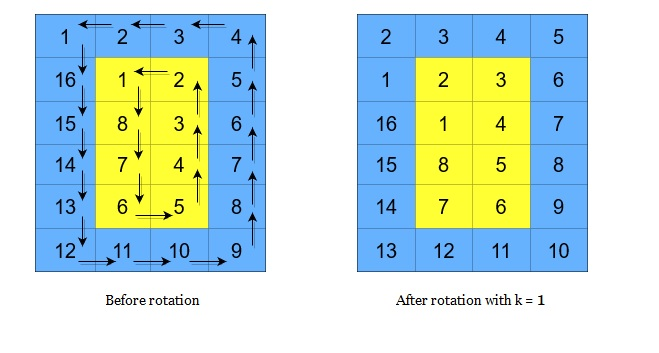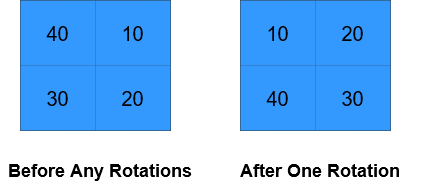```输入：grid = [[40,10],[30,20]], k = 1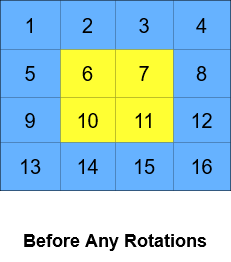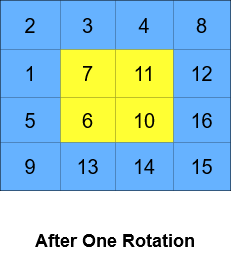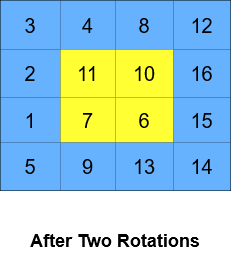```输入：grid = [[1,2,3,4],[5,6,7,8],[9,10,11,12],[13,14,15,16]], k = 2

```

• `m == grid.length`
• `n == grid[i].length`
• `2 <= m, n <= 50`
• `m``n` 都是 偶数
• `1 <= grid[i][j] <= 5000`
• `1 <= k <= 109`

[数组] [矩阵] [模拟]